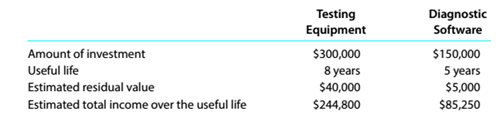Chapter 15, Problem 15.1E

Chapter
Section
Textbook Problem

Average rate of returnThe following data are accumulated by McDermott Motors Inc. evaluating two competingcapital investment proposals:Determine the expected average rate of return for each proposal.

To determine

Concept Introduction:

ARR:

Accounting Rate of Return (ARR) is the rate of return earned on the investment made in a project. ARR is calculated by dividing the Average Accounting profits by Average Investment.

The formula to calculate ARR is as follows:

ARR= Average Accounting profitsAverage Investment

To Calculate:

The Average Rate of return for each project

Explanation

The Average Rate of return for each project is calculated as follows:

... Testing Equipment Diagnostic Software Expected Total Income (A) $244,800$ 85,250 Number of years (B) 8

Still sussing out bartleby?

Check out a sample textbook solution.

See a sample solution

The Solution to Your Study Problems

Bartleby provides explanations to thousands of textbook problems written by our experts, many with advanced degrees!

Get Started